---===( A COMET'S TALE )===---Credit: Comet Halley as taken March 8, 1986 by W. Liller, Easter Island.

I. INTRODUCTION

Halley’s Comet is arguably one of the most famous comet known to men. There have been records of it dating back to 240 B.C., where Chinese observers spotted it. Last spotted in 1986, and with a period of 76 years, Halley's Comet is expected to be spotted again in 2061. Comets are sometimes referred to as “dirty snowballs”. This is because they are mostly black in color and are mainly made up of frozen gases, ice, and dust with a small amount of other solids embedded inside. When a comet nears the sun, the comet’s surface is heated up by the sun. This causes the release of gas and other particles from the comet surface which creates a tail behind the comet that is visible from far away. The orbit of Halley's Comet is highly eccentric and does not follow the orbits of normal planets. We shall further explore the orbit of Halley’s Comet using Kepler's Second Law, Kepler's Equation, Newton's Universal Law of Gravitation, and the Gravitational Slingshot.

Period: 76 years
Eccentricity: 0.967
Orbit size: 17.83AU
Perihelion distance: 0.587AU
Aphelion distance: 35.3AU

II. NEWTON'S UNIVERSAL LAW OF GRAVITATION

Newton's Universal Law of Gravitation states that all objects attract each other with a certain amount of gravitational force. This force is directly proportional to the mass of the object and inversely proportional to the square of their distances. The formula is given as: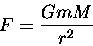As we can see from the formula, if the mass of an object doubles, the force will also double. If the distance doubles, the force will be reduced to a quarter of its original force. The program contained within the picture below helps illustrate this point.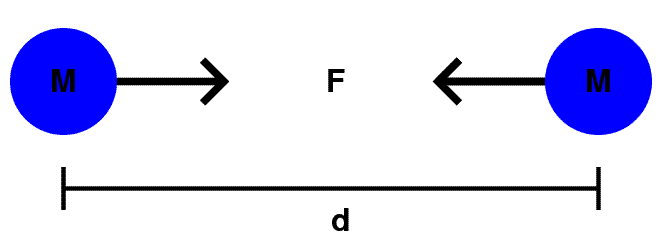In the case of planetary motion, since the comet is much smaller then the Sun, the mass of the Sun is approximately the same as the mass of the Sun and comet combined. In this situation, the comet doesn't exert enough gravitational force to move the Sun, but the Sun have enough gravitational force to move the comet. So only the comet only ends up moving.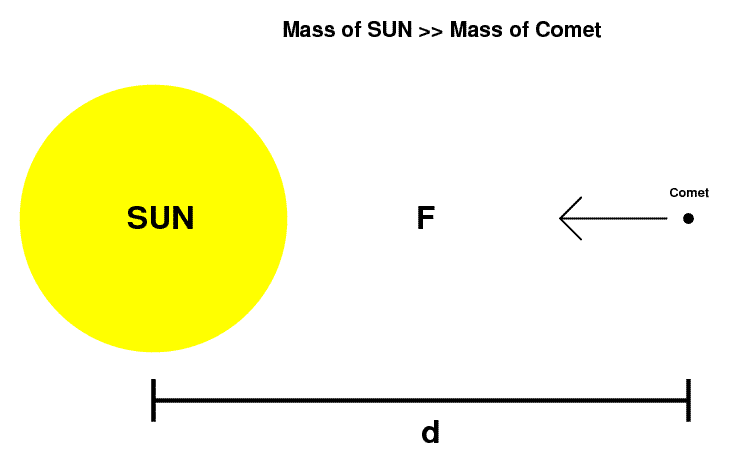As a result, Halley's Comet ends up orbiting and Sun, while the Sun remains stationary. But what exactly is the orbit of Halley's Comet?

III. KEPLER'S EQUATION & KEPLER'S SECOND LAW

Usually finding the position of a planet with respect to time is not that difficult if the orbit is circular. However, if the orbit is elliptical, finding the position becomes much harder. This is where Kepler's Equation comes in handy.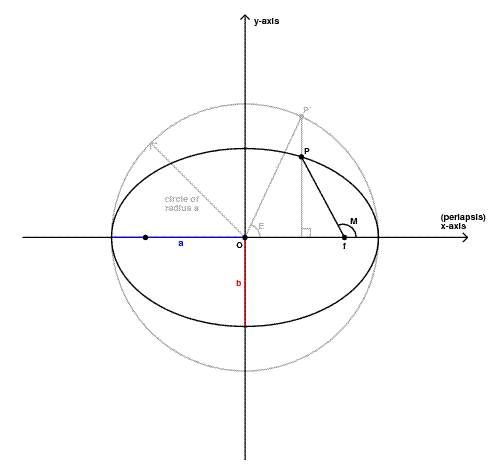Given that the orbit is elliptical, with semi-major axis a and semi-minor axis b, draw a circle from the origin with radius a. Assume that point P is the point where the planet is at a certain time t. Drawing a vertical line through P, we will intersect the circle. This intersection we will call P'. The angle that is formed when a line is drawn from P' to the origin is called E. E is called the eccentric anomaly. By finding E, we will be able to calculate the position P of the planet through Kepler's Equation.

Kepler's Equation:

M = E - e*(sin E)

where e is the eccentricity, and M is the mean anomaly.
The mean anomaly M is a parameterization of time and can be calculated by: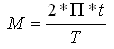where T is the period of orbit, and t is time since periapse.
Once E is found, the point P is just equals (a*cos E, b*sin E).

By using Kepler's Equation and the information we know about Halley's Comet (period and eccentricity) we can plot the position of Halley's Comet at different time intervals. But wait! Let's see if we can verify this method visually by using Kepler's Second Law.

Kepler's Second Law states that a line joining a planet and its stars sweeps out equal areas during equal intervals of time.

We will plot the position of Halley's Comet every 1 year for the duration of 5 years. The program contain within the picture below is an actual working example of the orbit of Halley's Comet around the Sun. The points are taken at one year intervals.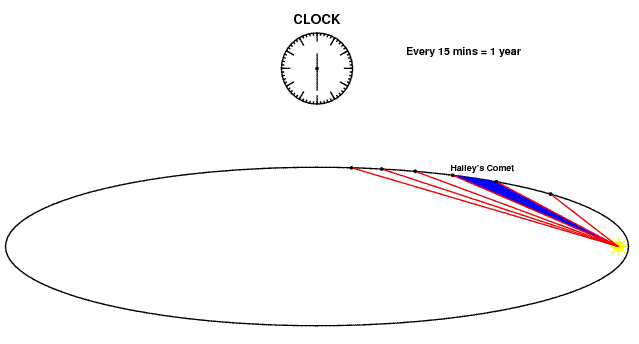Looks like Kepler's Second Law agrees with Kepler's Equation. So, is that all there is to the orbit of Halley's Comet? The answer is a resounding NO!

IV. GRAVITATIONAL SLINGSHOT

When a smaller planetary body like a comet, comes in close contact to a much larger planet, the comet gains speed from the planet's orbital speed. An analogy would be to roll a small steel ball (comet) towards a spinning magnet (planet). If done correctly, the steel ball would be pulled in by the magnet (Newton's Universal Law of Gravitation), and be thrown out at a faster speed then what the ball was initially going in with. This is because the steel ball (comet) gained speed from the spinning of the magnet (orbital speed).

Let's begin with the simplest example. If the planet has zero orbital speed, and the comet is approaching the planet with a speed V, it would be pulled in by its gravity, not gaining any additional speed, and exit with the same speed V but in the opposite direction it came from.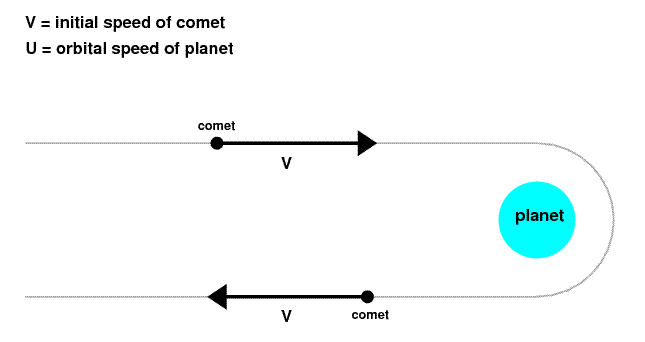If however the planet is spinning with orbital speed U, the comet would gain speed from the planet's orbital speed and exit at speed 2U+V.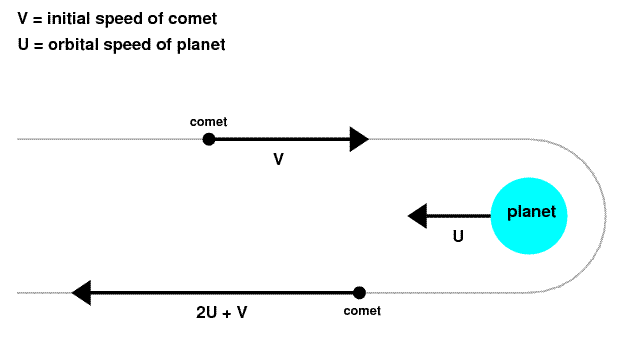Similarly, if a comet approaches the planet at velocity V1 and at an angle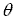, with zero planet orbital speed, the comet exits at velocity V2 where V2x = -V1*cos, and V2y = V1*sin.If however, the comet is entering at velocity V1 and the planet's orbital speed is U, the comet would gain velocity in the V2x direction and exit with velocity V2 where V2x = -V1*cos+ 2U, and V2y = V1*sin.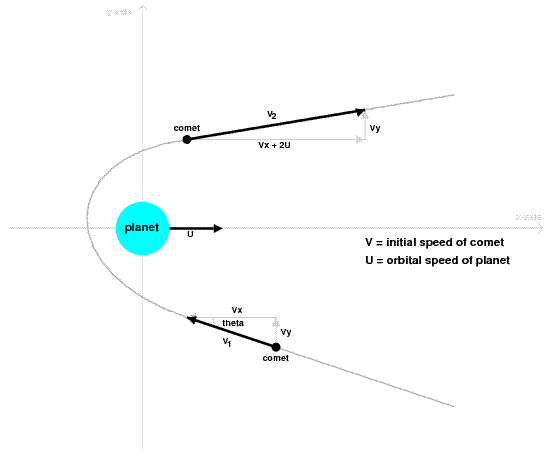This gain in comet's speed from a planet's orbital speed is called a Gravitational Slingshot. This will have an impact on the orbit of Halley's Comet as every time Halley's Comet comes in proximity with another spinning planet, it would gain speed from the planet and slingshot off at a different trajectory.

V. SUMMARY

By using Kepler's Second Law, Kepler's Equation, Newton's Universal Law of Gravitation, and the Gravitational Slingshot, I have tried to explain Halley's orbit. This list is by no means exhaustive. Another aspect in determining a comet's orbit would be that the comet loses some mass every time it approaches the sun. This is due to the release of gases and other solids from the surface being heated up by the sun. This change in mass would create a different gravitational force from the comet and therefore changing the comet's path. Though the orbit of Halley's Comet is not covered in its entirety, hopefully, this project has covered some of the major explanations of a comet's orbit.

VI. REFERENCES

MATH 308 Project
Created by: Clement Lim
41631037